# Latest word problems

#### Number of problems found: 823

• Pentagonal pyramidFind the volume and surface of a regular pentagonal pyramid with a base edge a = 12.8 cm and a height v = 32.1 cm.
• IQ Intelligence quotientIntelligence quotient (IQ) is a standardized score used as the output of standardized intelligence psychological tests to quantify a person's intelligence with the rest of the population (respectively to a given group). Intelligence has an approximately n
• Calculate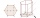Calculate the surface of a regular eleven-sided prism, if the content of its base is 58cm2, the edge of the base is 6cm long, the height of the prism is 21cm
• Three colors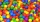Find the probability that 3 balls of the same color will be drawn from fate with 10 white, 10 red, and 10 blue balls.
• Coke and coal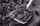20.1 tons of coke is produced from 30 tons of black coal. How much coke is made from 1 kilogram of coal?
• Assembly timeThe assembly time for the toy follows a normal distribution with a mean of 75 minutes and a standard deviation of 9 minutes. The company closes at 5 pm every day. If one starts assembling at 4 pm what is the probability that he will finish before the comp
• Alopecia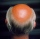Medical literature indicates that 45% of men suffer from alopecia. For random sample of 8 men, calculate the probability that: (a) exactly four men suffer from alopecia. (b) at most two men suffer from alopecia.
• Secondary school1/2 of the pupils want to study at the secondary school, 1/4 at the apprentice, 1/6 at the grammar school 3 pupils do not want to study. How many students are in the class?
• Convalescent homes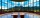In 270 convalescent homes, 94,270 vacationers spent part of the holiday a year. On average, how many vacationers per convalescent home?
• Isosceles trapezoidFind the area of an isosceles trapezoid with bases of 8cm and 72mm. The height of the trapezoid is equal to three-quarters of the longer base.
• The recommendedThe recommended price of the novel "Laughing Sun" is 285 SKK. The bookseller bought 60 pieces of the novel at the wholesale store and paid 82% of the recommended price (18% is his profit for selling books). For the recommended price, he sold 55 pieces ofThe regular quadrilateral pyramid has a base edge a = 1.56 dm and a height h = 2.05 dm. Calculate: a) the deviation angle of the sidewall plane from the base plane b) deviation angle of the side edge from the plane of the base
• Wooden bowls20 wooden bowls in the shape of a truncated cone should be painted on the outside and inside with wood varnish. We need 0.1 l of paint to paint 200 cm2. How many liters of paint do we have to buy if the bowls are 25 cm high, the bottom of the bowl has a d
• From plasticine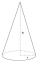Michael modeled from plasticine a 15 cm high pyramid with a rectangular base with the sides of the base a = 12 cm and b = 8 cm. From this pyramid, Janka modeled a rotating cone with a base diameter d = 10 cm. How tall was Janka's cone?
• Two vases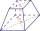Michaela has two vases in her collection. The first vase has the shape of a cone with a base diameter d = 20 cm; the second vase has the shape of a truncated cone with the diameter of the lower base d1 = 25 cm and with the diameter of the upper base d2 =
• DecibelBy what percentage does the sound intensity increase if the sound intensity level increases by 1 dB?
• Cuboid diagonalsThe cuboid has dimensions of 15, 20 and 40 cm. Calculate its volume and surface, the length of the body diagonal and the lengths of all three wall diagonals.
• AquariumHow many liters of water can fit in an aquarium measuring 30, 15 and 20 cm?
• This year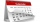This year 2020 is a leap year. How many leap years there had been be since 1904?
• The sum 6The sum of the 17 different natural numbers is 153. Determine the sum of the two largest ones.

Do you have an interesting mathematical word problem that you can't solve it? Submit a math problem, and we can try to solve it.

We will send a solution to your e-mail address. Solved examples are also published here. Please enter the e-mail correctly and check whether you don't have a full mailbox.

Please do not submit problems from current active competitions such as Mathematical Olympiad, correspondence seminars etc...